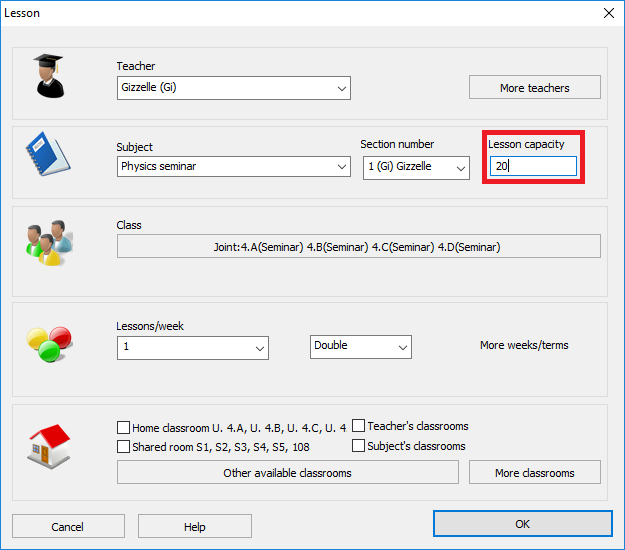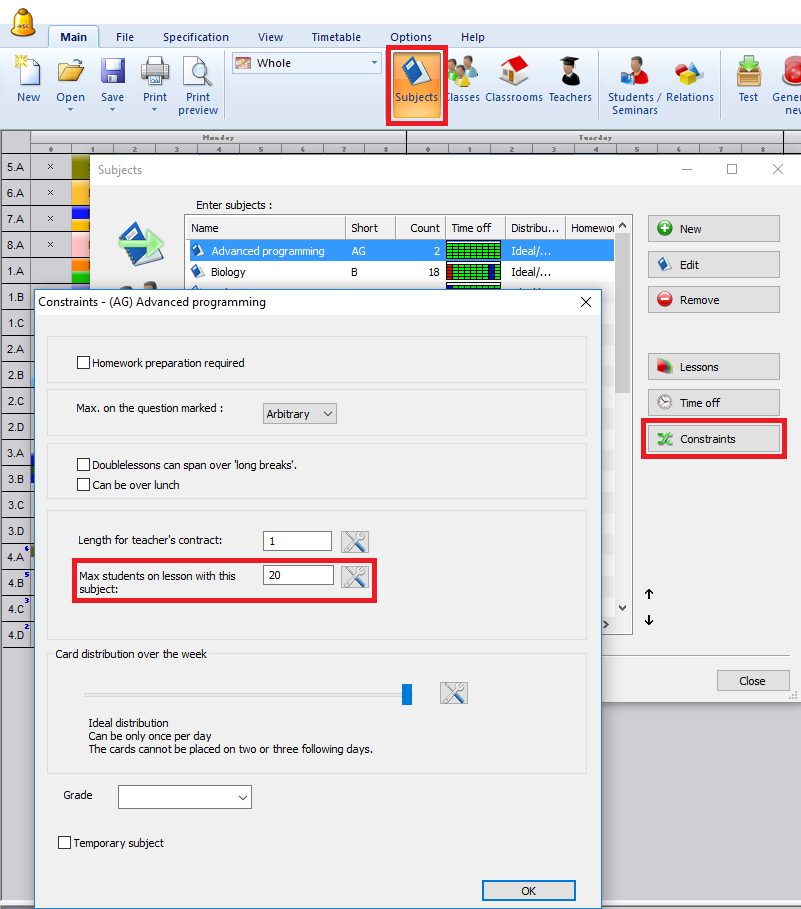#### aSc Raspored sati - Online pomoćaSc EduPage - Online HelpaSc Video HelpaSc Raspored sati - Seminars/Courses

Max students for certain seminar lesson
Ovaj članak je dostupan na:

It is possible to input max number of students that can be assigned to given seminar (section). You can input it in "Lesson" dialog in "Lesson capacity" field:Note: you can input both minimum and maximum capacity: if you input 10-20 this means that all solution where there are between 10 and 20 students are considered correct for the generator.
If you input only 20 - then this number indicates maximum. The minimum is calculated as number of students / number of sections +- 10%. This 10% can be specified in the seminar/settings section.

It is also possible to input this for all lessons of some subject in subject constraints:Note: Value for subject is used only for lessons where "Lesson capacity" field is left blank. If you input "Lesson capacity" in lesson, then value from subject is ignored for that lesson.Ovaj članak je pregledan 56555 puta.44393 / 56555Prošli članak Povratak na aSc Raspored sati help index Sljedeći članak